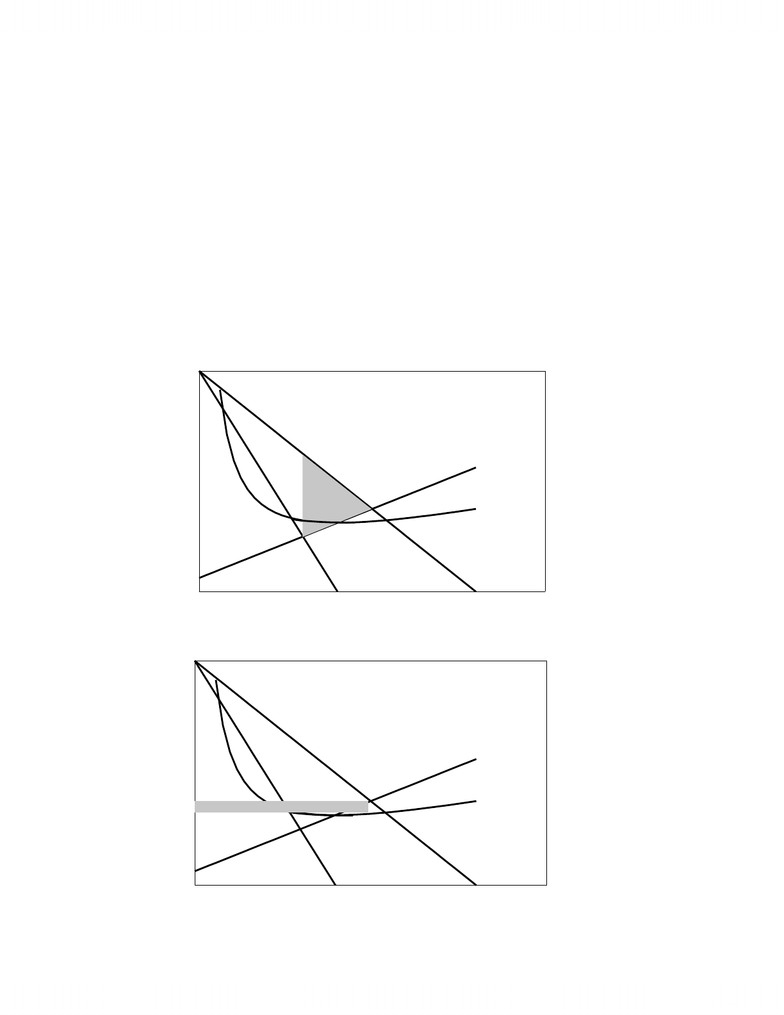Class Notes (1,100,000)
CA (620,000)
UTSG (50,000)
ECO (2,000)
A (10)
Lecture

ECO204Y1 Lecture Notes - Externality, Pareto Efficiency, Allocative Efficiency

Department
Economics
Course Code
ECO204Y1
Professor
A

This preview shows pages 1-2. to view the full 8 pages of the document.Principles of Economics: Efficiency
EFFICIENCY
Definition: Productive Efficiency is optimal output from given resources or minimal cost for a
given output.
We have already seen the concept of productive efficiency in our discussion of Production
Possibilities.
Definition: Allocative Efficiency is the optimal allocation of resources
Allocative Efficiency implies not only the optimal output from given resources (Productive
Efficiency) but also the optimal output desired by society. Allocative Efficiency is the output that
maximizes the net social benefit of society, i.e., maximizes the difference between total benefit and
total cost to society.
Maximum Net Social Benefit => P = MC
Maximum Net Social Benefit implies the output where the benefit to society of an additional
unit of output equals the cost to society of an additional unit of output. It is the output where
Marginal Benefit equals Marginal Cost (MB = MC). Since we measure the marginal benefit by
willingness to pay, the market Demand for a commodity measures the Marginal Benefit of a
commodity. We can therefore say that the allocation of resources is optimal at the output where
Price equals Marginal Cost (P = MC).
Pareto Efficient Allocation (Pareto Optimality) => P = MC
Definition: Pareto Optimality is the allocation of resources where no person is worse off from
trading (allocation) and any addition trade (allocation) will make some person worse off.
The Pareto Optimality condition is also satisfied by the output where P = MC.
- 1 -

Only pages 1-2 are available for preview. Some parts have been intentionally blurred.Principles of Economics: Efficiency
Efficiency Loss (aka Welfare Loss or Deadweight Loss)
We measure the efficiency loss of an output Qo by the difference between Price and Marginal
Cost (Supply) between the output Qo and optimal output at P = MC. It is the difference between
Demand and Marginal Cost (Supply) between given output and optimal output. We will see that
this is equivalent to the net loss of consumers’ surplus and producers’ surplus at the output relative
to optimal output.
Competition => Optimum Allocation (Allocation Efficiency, 0 Efficiency Loss)
Since firms and households are price-takers in a competitive market, competitive market
equilibrium implies that P = MC, which implies that competitive markets give the optimal
allocation of resources.
Monopoly Regulation
Monopolies create efficiency loss by reducing output below competitive output and
increasing price above competitive price. Governments cannot regulate monopolies through
taxation, though, since per unit taxes decrease monopoly output by shifting up Marginal Cost and
fixed taxes do not change monopoly output since marginal cost doesn’t change.
Governments attempt to eliminate the efficiency loss caused by a monopoly by requiring that
a ‘normal’ monopoly produce the output where Price equals Marginal Cost (P = MC) and that a
natural monopoly produce the output where Price equals Average Cost.
1. Marginal Cost Pricing
The principal concern of government regulation of monopoly is to eliminate efficiency loss
not to eliminate monopoly profits. Marginal Cost Pricing (P = MC) as the requirement that the
monopoly produce the output where Price equals Marginal Cost is therefore the preferred option
for government regulation. The upper diagram below shows the efficiency loss due to a normal
- 2 -
You're Reading a Preview

Unlock to view full version

Only pages 1-2 are available for preview. Some parts have been intentionally blurred.Principles of Economics: Efficiency
monopoly above and the lower diagram below shows the output, price, and economic profit and
loss of the monopoly given marginal cost pricing. You will note that marginal cost pricing results
in 0 efficiency loss but still leaves the monopoly with an economic profit. The monopoly can still
exist with greater than normal profits while society achieves optimal allocation of resources. (This
is only the short-run optimum allocation since firms would enter the competitive market in the
long-run so that 0 efficiency loss would occur where price equals marginal cost at minimum
average cost)
0 10 20 30 40 50
0
20
40
60
80 P, Cost/unit, MR
Q
Demand
MR
Qm
Pm MC
Efficiency
Loss
AC
P = 80 - 2Q; TC = Q
2
/2+5Q+200 => AC = Q/2 + 5 + 200/Q and MC = Q + 5
P = 80 - 2Q; TC = Q
2
/2+5Q+200 => AC = Q/2 + 5 + 200/Q and MC = Q + 5
0 10 20 30 40 50
0
20
40
60
80 P, Cost/unit, MR
Q
Demand
MR
Q
MC
P
MC
MC
AC
Economic Profit
- 3 -
You're Reading a Preview

Unlock to view full version